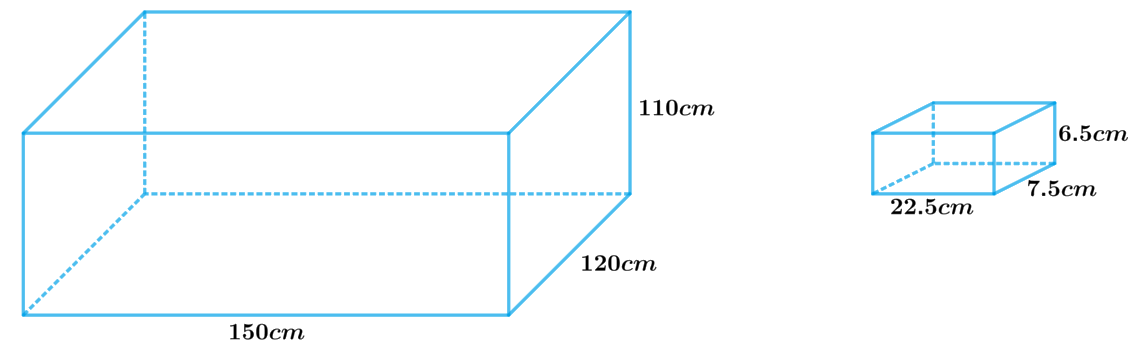# Ex.13.5 Q3 Surface Areas and Volumes Solution - NCERT Maths Class 10

Go back to  'Ex.13.5'

## Question

A cistern, internally measuring $$150\,\rm{cm}\, \times$$ $$120\,\rm{cm}$$ $$×$$ $$110\,\rm{cm,}$$ has $$129600$$ $$\rm cm^3$$of water in it. Porous bricks are placed in the water until the cistern is full to the brim. Each brick absorbs one-seventeenth of its own volume of water. How many bricks can be put in without overflowing the water, each brick being $$22.5\,\rm{cm}\,×$$ $$7.5\,\rm{cm}\, ×$$ $$6.5\,\rm{cm}$$ ?

## Text Solution

What is Known?

A cistern internally measuring $$150\,\rm{cm }\,\times$$  $$120\,\rm{cm }\, \times$$ $$110\,\rm{cm}$$ has $$129600$$ $$\rm{c{m^3}}$$ of water in it. Each brick is of dimensions $$22.5 \times 7.5 \times 6.5\rm{cm}$$ and can absorbs one-seventeenth of its own volume of water.

What is Unknown?

Number of bricks which can be put in the cistern without overflowing the water.

Reasoning:Logically, the bricks have to occupy the space left in the cistern which is not occupied by water left in the cistern, after being absorbed by the bricks.

Therefore,

Internal volume of cistern $$=$$ (Volume of water in the cistern $$–$$ Volume of water absorbed by bricks) $$+$$ Volume of bricks

Internal volume of cistern $$=$$ Volume of water in cistern $$-$$ \begin{align}\frac{1}{{17}}\end{align} $$\times$$ Volume of  bricks $$+$$ volume of bricks

Hence,

Internal volume of cistern $$=$$ Volume of water in the cistern\begin{align} + \frac{{16}}{{17}}\end{align} volume of brick

Since the dimensions of the cistern and the brick are known which are cuboidal in shape

We will find the volume by using formula;

Volume of the cuboid $$= lbh$$

where $$l, b$$ and $$h$$ are length breadth and height of the cuboid respectively.

Steps:

Internal volume of the cistern \begin{align} = 150cm\! \times\! 120cm \!\times\! 110cm = 1980000c{m^3}\end{align}

Volume of water in the cistern $$= 129600c{m^3}$$

Volume of one brick

\begin{align}& = 22.5cm \times 7.5cm \times 6.5cm \\&= 1069.875c{m^3}\end{align}

Let the required number of bricks be $$‘x’$$

Volume of $$x$$ bricks \begin{align} = x \times 1069.875c{m^3}\end{align}

Internal volume of cistern $$=$$ Volume of water in the cistern $$+ \frac{{16}}{{17}}$$ volume of $$x$$ bricks

\begin{align}1980000c{m^3} &= 129600c{m^3} + \frac{{16}}{{17}} \times x \times 1096.875c{m^3}\\ x &= \frac{{\left( {1980000c{m^3} - 129600c{m^3}} \right) \times 17}}{{16 \times 1096.875c{m^3}}}\\ &= \frac{{1850400c{m^3} \times 17}}{{16 \times 1096.875c{m^3}}}\\ &= 1792.41\end{align}

Since the number of bricks should be a whole number, whole part of $$x$$ should be considered as the answer. Rounding off $$x$$ to the next whole number should not be considered as it will lead to overflowing of tank, if that extra fractional part is added to the cistern.

Therefore, number of bricks which can be put in the cistern without overflowing the water is $$1792.$$

Learn from the best math teachers and top your exams

• Live one on one classroom and doubt clearing
• Practice worksheets in and after class for conceptual clarity
• Personalized curriculum to keep up with school